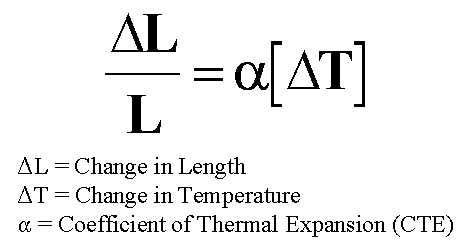A# coefficient of linear expansionThe coefficient of linear expansion is the change in length of a specimen one unit long when its temperature is changed by one degree (see linear expansion).

Different materials expand by different amounts. Just how much a particular material expands depends on the forces of cohesion between neighboring atoms or molecules. If these forces are strong, the material can only expand slightly for a given rise in temperature. A metal such as lead which has a low melting point and is relatively easily compressed, expands a great deal for a given rise in temperature.

In order to compare the abilities of different solids to expand on heating it is necessary to refer to a table of coefficients of linear expansion. It doesn't matter what the unit of length used happens to be – whether it is a foot or a meter, an inch or a centimeter – providing the change in length is measured in the same units. What does matter, however, is whether the change in temperature is one degree Celsius or one degree Fahrenheit. Coefficients of linear expansion are usually given in "per °C", though some tables give them in "per °F". Since one degree Fahrenheit is equal to 5/9 of one degree Celsius the expansion for a rise of 1°C will be only 5/9 as much as for a rise of 1°C. For example, the coefficient of linear expansion of brass is 0.0000189 per °C. To convert to the Fahrenheit system, the coefficient is multiplied by 5/9 – 0.0000189 × 5/9 = 0.0000105 per °F. To convert from "per °F" to "per °C", multiply by 9/5.

Coefficients of linear expansion are represented by the Greek letter α (alpha) and are stated for ordinary room temperature.

Coefficients of linear expansion
for some common solids
Material (per °C)
aluminum 0.000025
brass 0.0000189
copper 0.000017